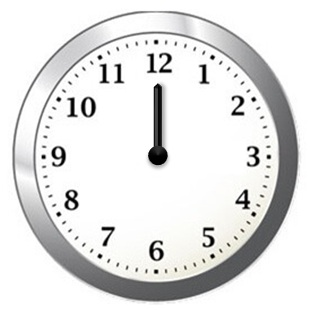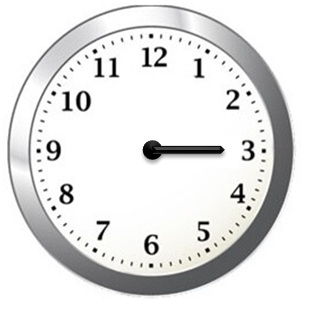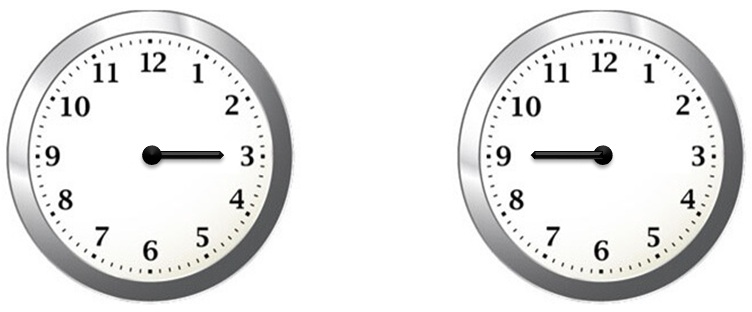Revolution in a clock

Chapter 5 Class 6 Understanding Elementary Shapes
Concept wiseSuppose we are at 12,

And we rotate right angleSo, we will be at 3

Since right angle makes 1/4 th of the complete rotation.

Hours rotated in right angle = 12/4

= 3

If we go from 3 to 9,We have turned 1/2 revolution

i.e. 2 × 1/4 revolution

i.e. 2 right angles

So, in a clock

12 hours = 1 revolution

1 hour = 1/12 revolution

3 hours = 3/12 = 1/4 revolution

6 hours = 6/12 = 1/2 revolution

So,

Full revolution  = 12 hours

Right angle = 3 hours

Straight angle = 6 hours

Learn in your speed, with individual attention - Teachoo Maths 1-on-1 Class